# GRE Subject Test: Physics : Diffraction

## Example Questions

### Example Question #1 : Diffraction

A beam of light, with wavelength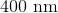, is normally incident on a transmission diffraction grating. With respect to the incident beam, the first order diffraction maximum occurs at an angle of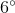. What is the number of slits per centimeter on the grating?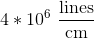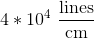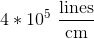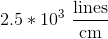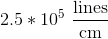Explanation:

The equation describing maxima of a diffraction grating is: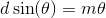Where d is the separation of slits, which can also be expressed as: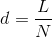Substituting d into the first equation and making the small angle approximation, one can solve for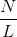(lines per length):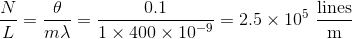Note that the angle had to be converted from degrees to radians. Finally, the question asks for lines per centimeter, not meter, so the answer becomes.

### All GRE Subject Test: Physics Resources